# RD Sharma Solutions For Class 7 Maths Exercise 4.2 Chapter 4 Rational Numbers

The PDF of RD Sharma Solutions for Class 7 Exercise 4.2 of Chapter 4 Rational Numbers are given below. Learners can access and download the PDF for free. The solutions to this exercise come with detailed explanations structured by our expert teachers that further makes learning and understanding of concepts an easy task. RD Sharma Solutions for Class 7 textbook includes the concepts in a detailed and step by step way to help the students understand in a better way. This exercise primarily deals with the properties of rational numbers. It also includes equivalent rational numbers defined as if p/q is a rational number and m is non zero integer, then ‘p × m/ q × m’ is a rational number equivalent to p/q. To learn more about this download the PDF is given below.

## Download the PDF of RD Sharma Solutions For Class 7 Maths Chapter 4 – Rational Numbers Exercise 4.2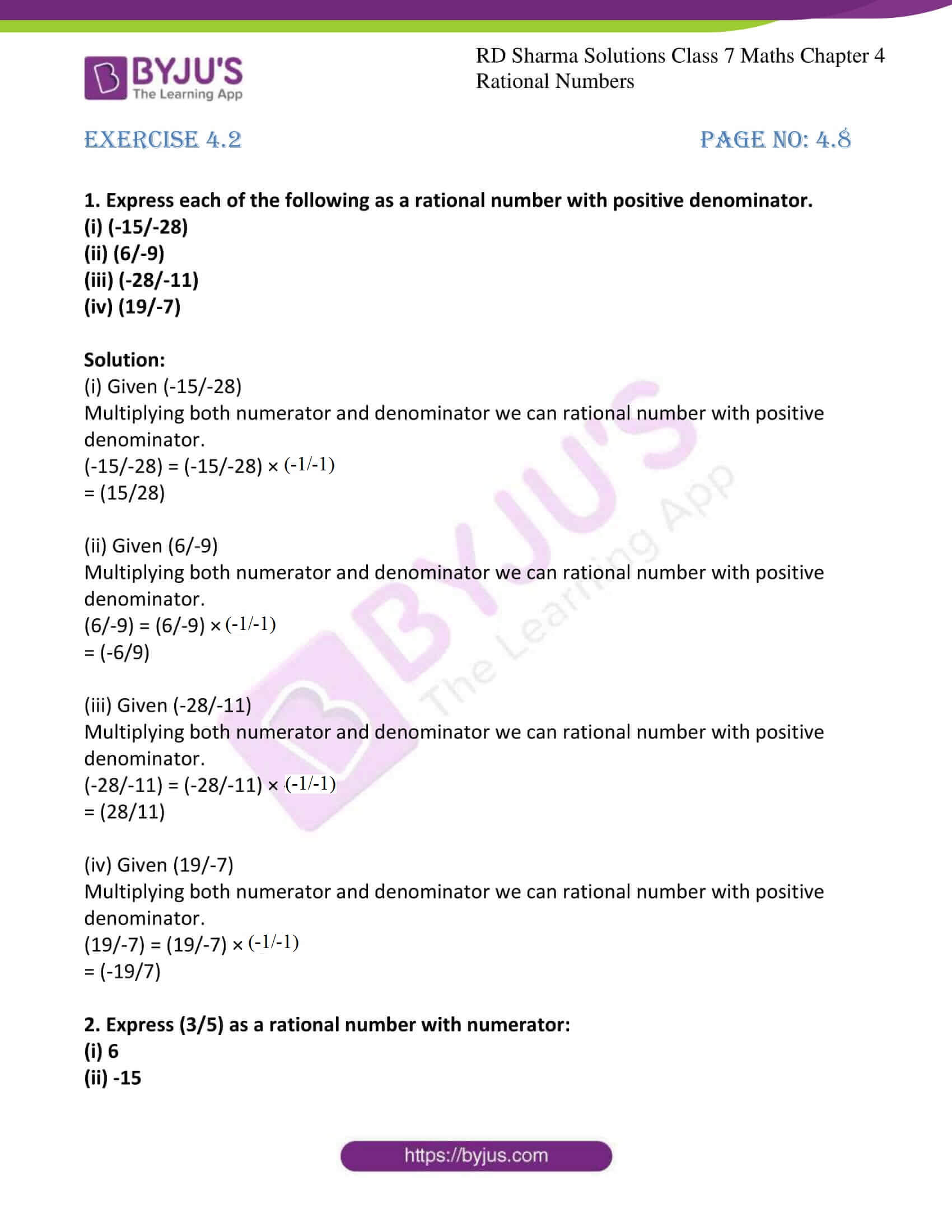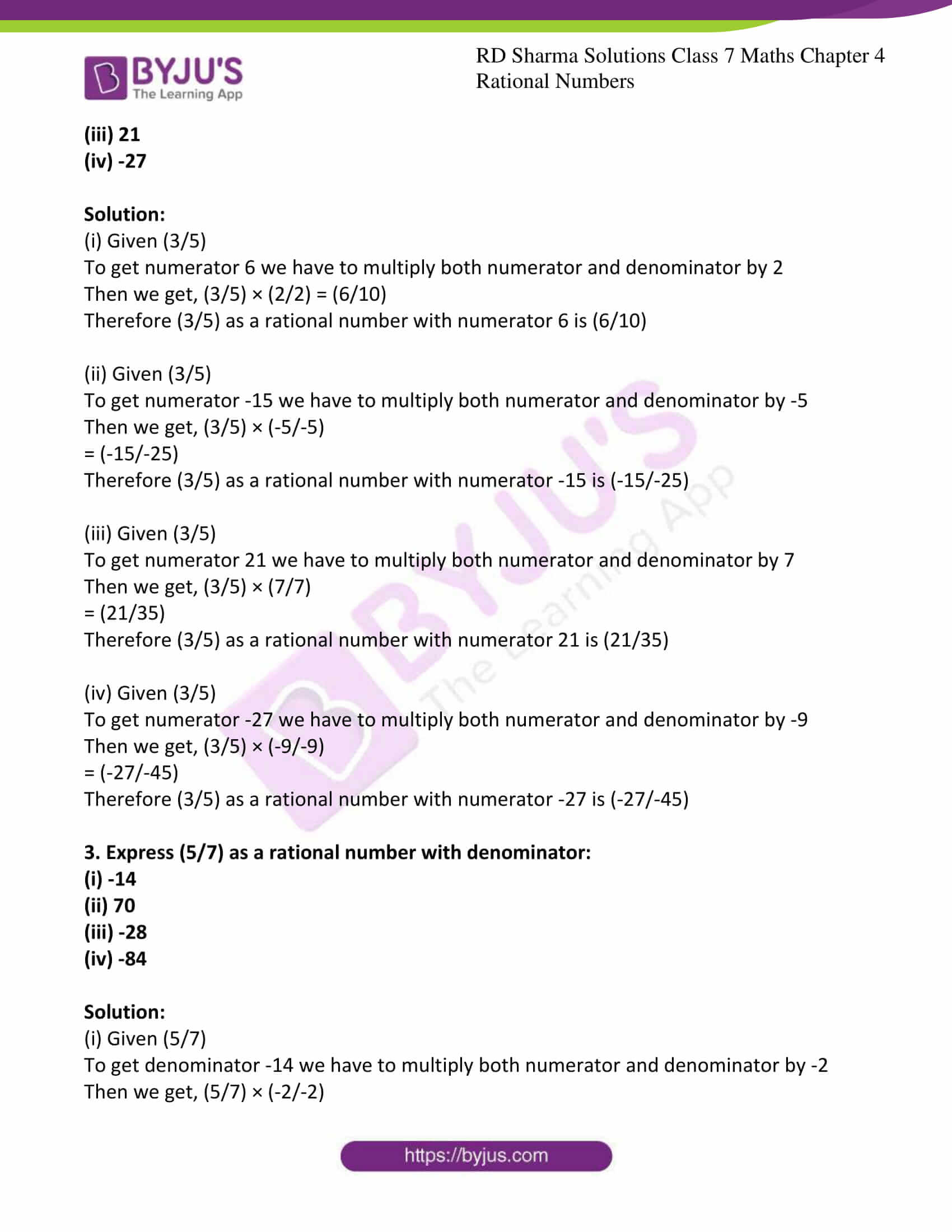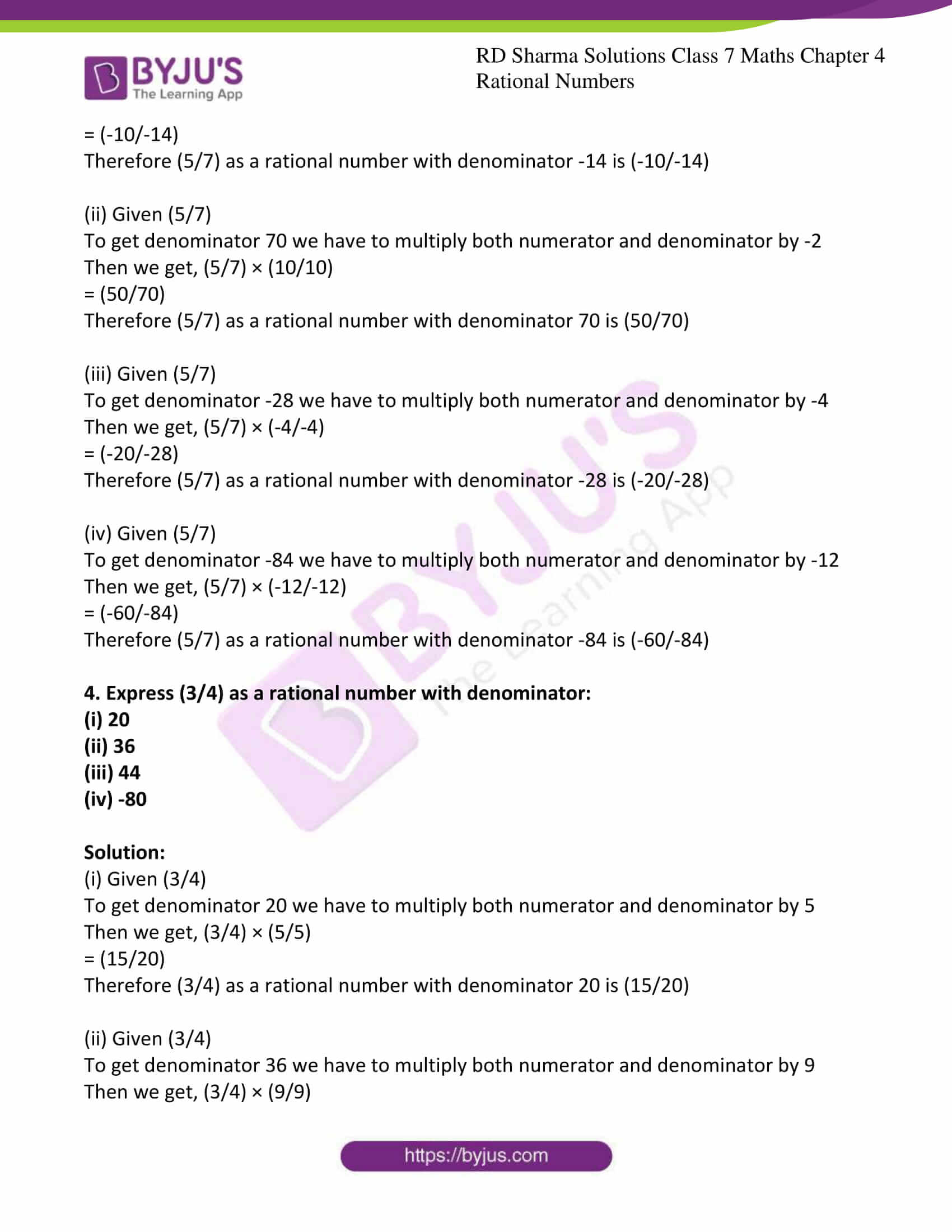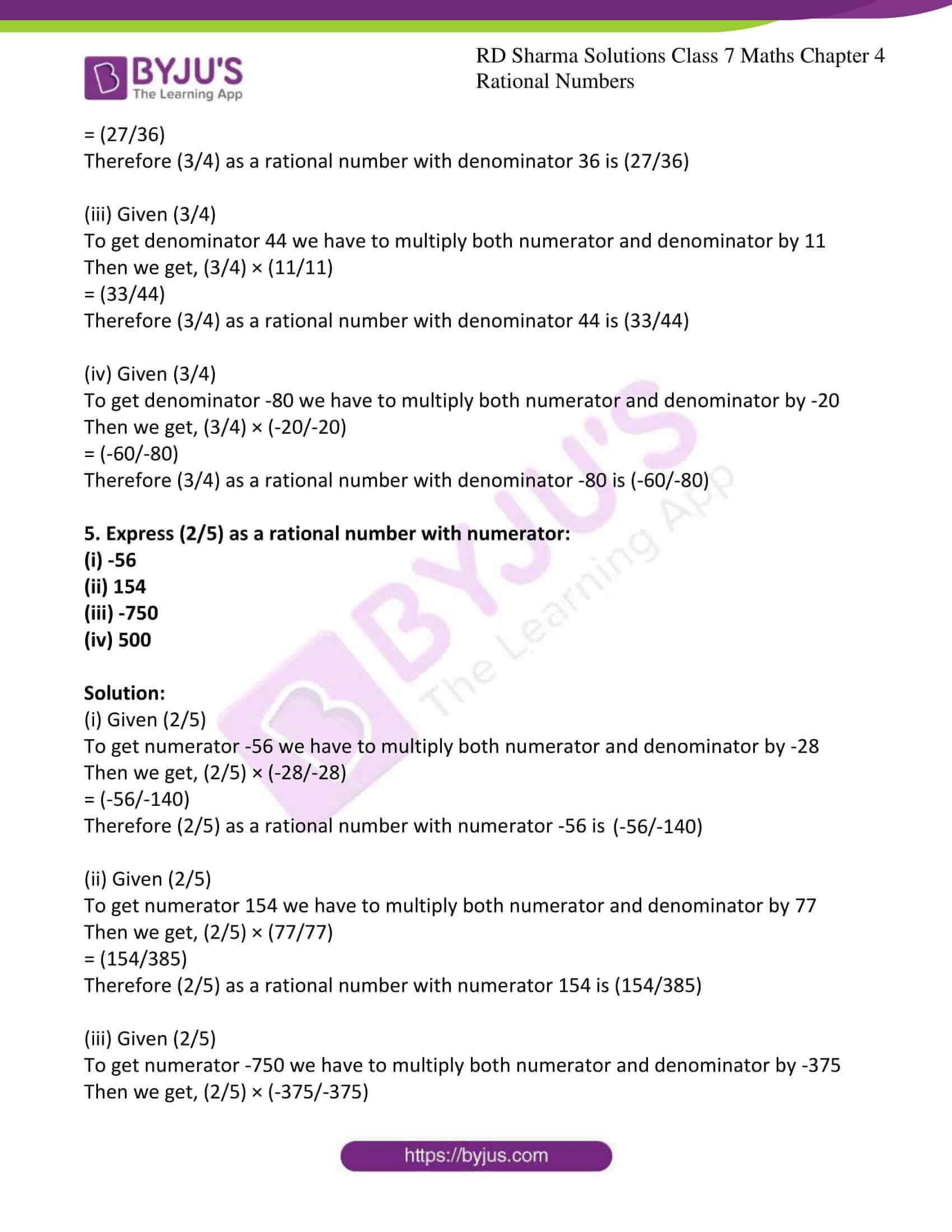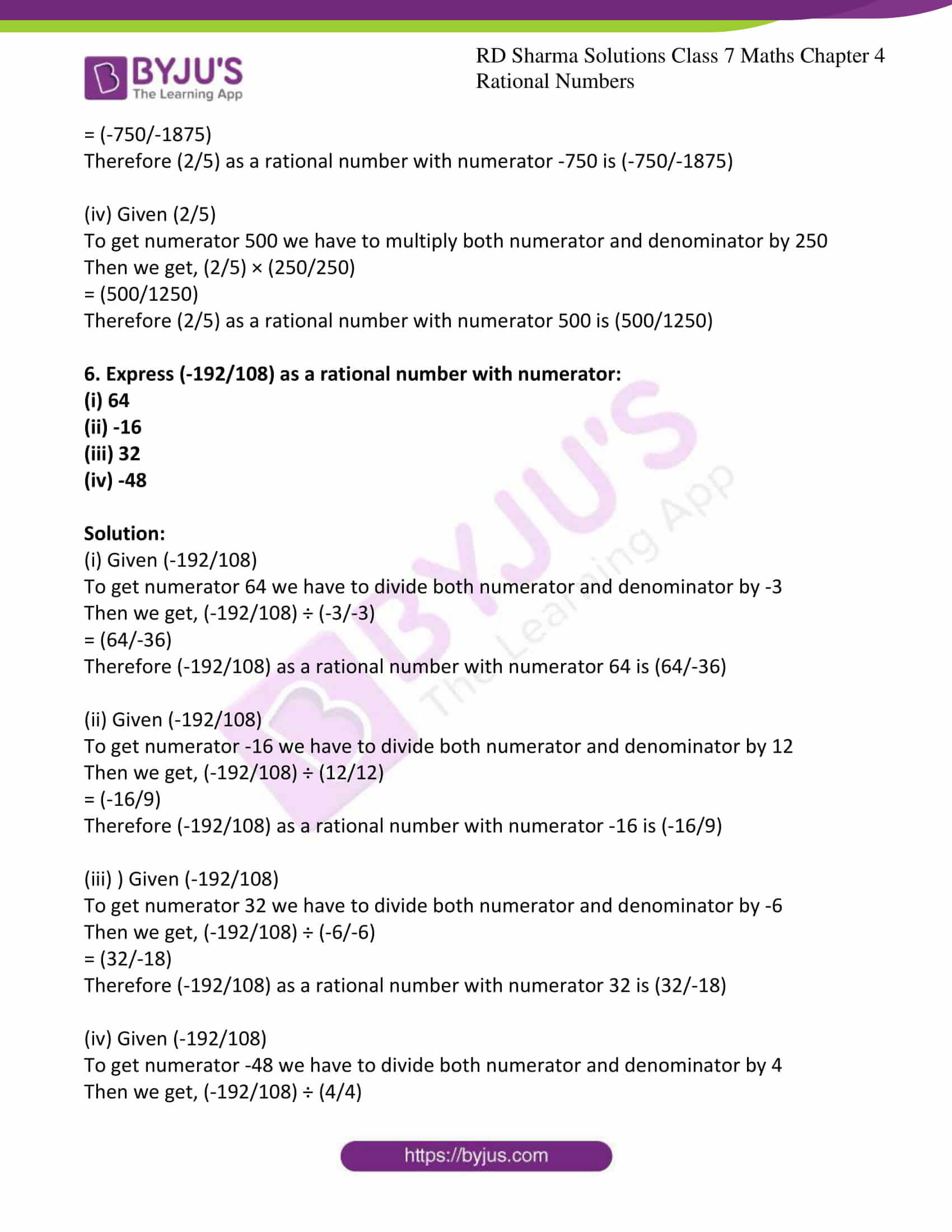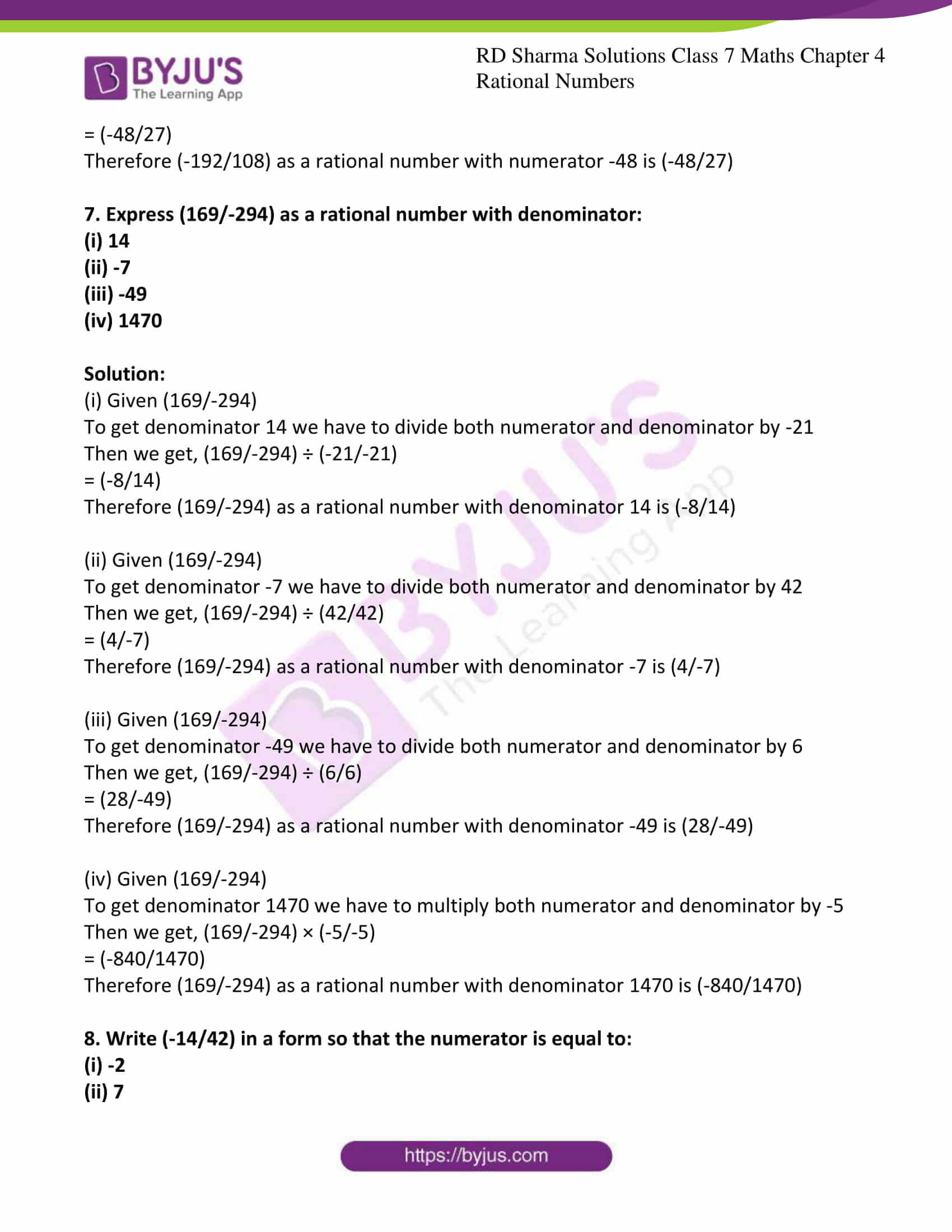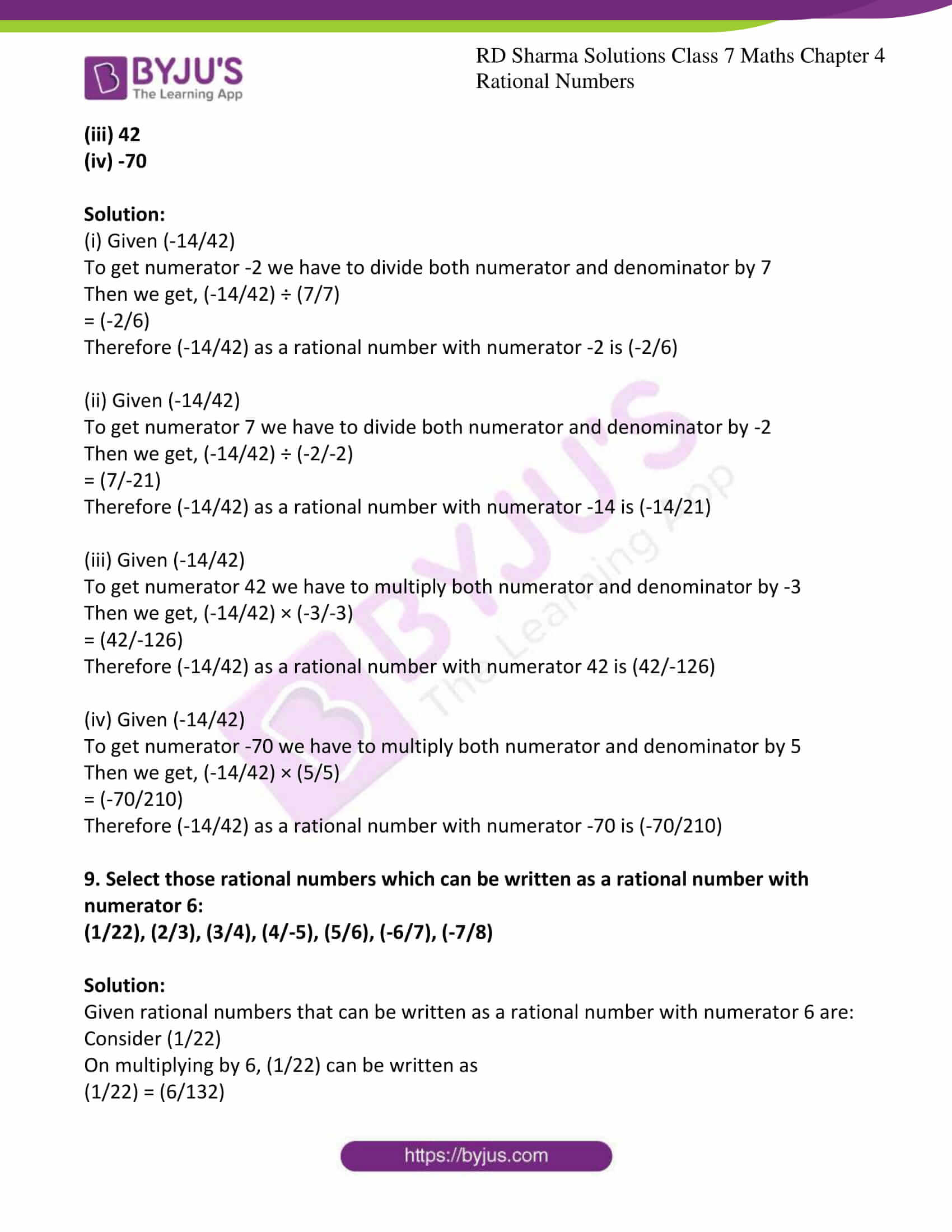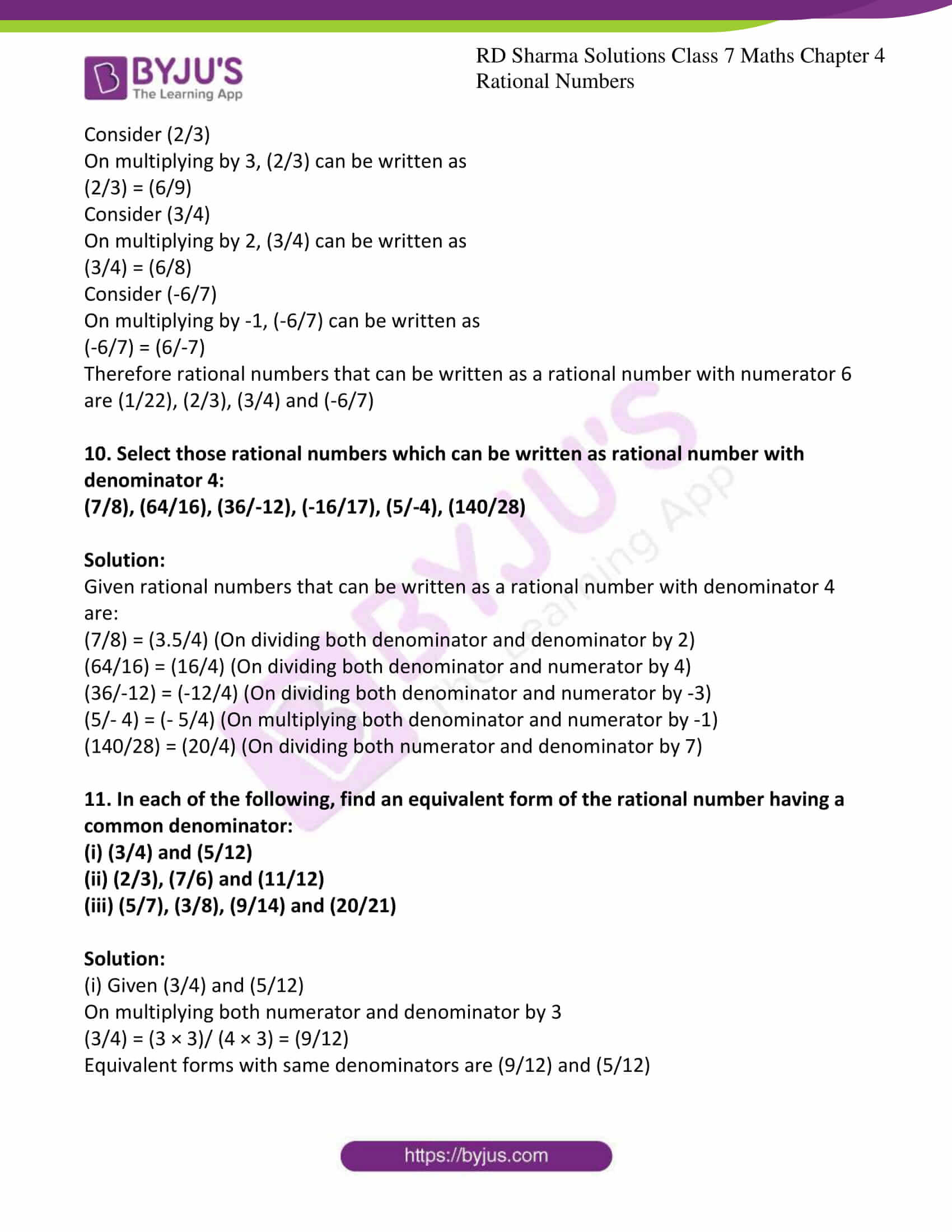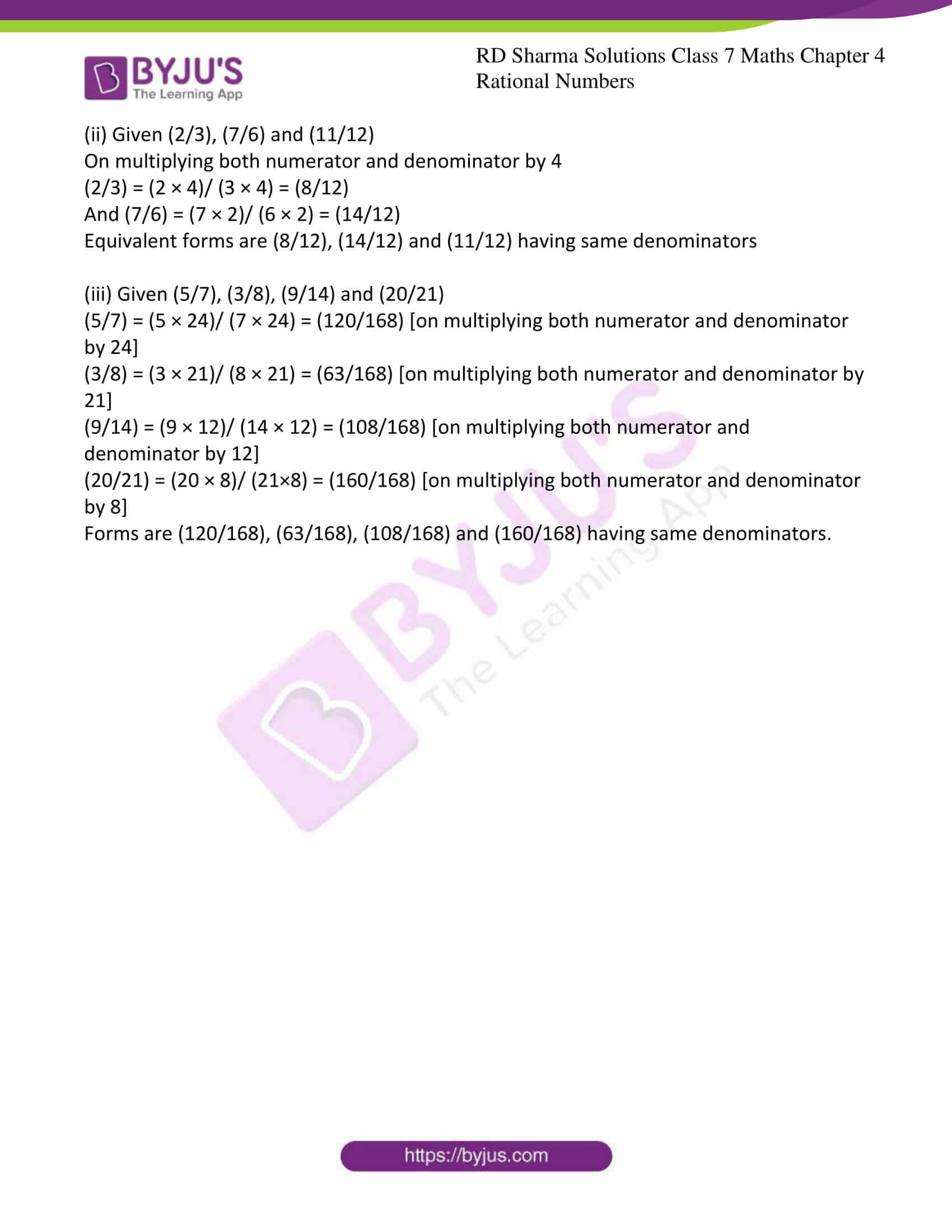### Access answers to Maths RD Sharma Solutions For Class 7 Chapter 4 – Rational Numbers Exercise 4.2

1. Express each of the following as a rational number with positive denominator.

(i) (-15/-28)

(ii) (6/-9)

(iii) (-28/-11)

(iv) (19/-7)

Solution:

(i) Given (-15/-28)

Multiplying both numerator and denominator we can rational number with positive denominator.

(-15/-28) = (-15/-28) × (-1/-1)

= (15/28)

(ii) Given (6/-9)

Multiplying both numerator and denominator we can rational number with positive denominator.

(6/-9) = (6/-9) × (-1/-1)

= (-6/9)

(iii) Given (-28/-11)

Multiplying both numerator and denominator we can rational number with positive denominator.

(-28/-11) = (-28/-11) × (-1/-1)

= (28/11)

(iv) Given (19/-7)

Multiplying both numerator and denominator we can rational number with positive denominator.

(19/-7) = (19/-7) × (-1/-1)

= (-19/7)

2. Express (3/5) as a rational number with numerator:

(i) 6

(ii) -15

(iii) 21

(iv) -27

Solution:

(i) Given (3/5)

To get numerator 6 we have to multiply both numerator and denominator by 2

Then we get, (3/5) × (2/2) = (6/10)

Therefore (3/5) as a rational number with numerator 6 is (6/10)

(ii) Given (3/5)

To get numerator -15 we have to multiply both numerator and denominator by -5

Then we get, (3/5) × (-5/-5)

= (-15/-25)

Therefore (3/5) as a rational number with numerator -15 is (-15/-25)

(iii) Given (3/5)

To get numerator 21 we have to multiply both numerator and denominator by 7

Then we get, (3/5) × (7/7)

= (21/35)

Therefore (3/5) as a rational number with numerator 21 is (21/35)

(iv) Given (3/5)

To get numerator -27 we have to multiply both numerator and denominator by -9

Then we get, (3/5) × (-9/-9)

= (-27/-45)

Therefore (3/5) as a rational number with numerator -27 is (-27/-45)

3. Express (5/7) as a rational number with denominator:

(i) -14

(ii) 70

(iii) -28

(iv) -84

Solution:

(i) Given (5/7)

To get denominator -14 we have to multiply both numerator and denominator by -2

Then we get, (5/7) × (-2/-2)

= (-10/-14)

Therefore (5/7) as a rational number with denominator -14 is (-10/-14)

(ii) Given (5/7)

To get denominator 70 we have to multiply both numerator and denominator by -2

Then we get, (5/7) × (10/10)

= (50/70)

Therefore (5/7) as a rational number with denominator 70 is (50/70)

(iii) Given (5/7)

To get denominator -28 we have to multiply both numerator and denominator by -4

Then we get, (5/7) × (-4/-4)

= (-20/-28)

Therefore (5/7) as a rational number with denominator -28 is (-20/-28)

(iv) Given (5/7)

To get denominator -84 we have to multiply both numerator and denominator by -12

Then we get, (5/7) × (-12/-12)

= (-60/-84)

Therefore (5/7) as a rational number with denominator -84 is (-60/-84)

4. Express (3/4) as a rational number with denominator:

(i) 20

(ii) 36

(iii) 44

(iv) -80

Solution:

(i) Given (3/4)

To get denominator 20 we have to multiply both numerator and denominator by 5

Then we get, (3/4) × (5/5)

= (15/20)

Therefore (3/4) as a rational number with denominator 20 is (15/20)

(ii) Given (3/4)

To get denominator 36 we have to multiply both numerator and denominator by 9

Then we get, (3/4) × (9/9)

= (27/36)

Therefore (3/4) as a rational number with denominator 36 is (27/36)

(iii) Given (3/4)

To get denominator 44 we have to multiply both numerator and denominator by 11

Then we get, (3/4) × (11/11)

= (33/44)

Therefore (3/4) as a rational number with denominator 44 is (33/44)

(iv) Given (3/4)

To get denominator -80 we have to multiply both numerator and denominator by -20

Then we get, (3/4) × (-20/-20)

= (-60/-80)

Therefore (3/4) as a rational number with denominator -80 is (-60/-80)

5. Express (2/5) as a rational number with numerator:

(i) -56

(ii) 154

(iii) -750

(iv) 500

Solution:

(i) Given (2/5)

To get numerator -56 we have to multiply both numerator and denominator by -28

Then we get, (2/5) × (-28/-28)

= (-56/-140)

Therefore (2/5) as a rational number with numerator -56 is (-56/-140)

(ii) Given (2/5)

To get numerator 154 we have to multiply both numerator and denominator by 77

Then we get, (2/5) × (77/77)

= (154/385)

Therefore (2/5) as a rational number with numerator 154 is (154/385)

(iii) Given (2/5)

To get numerator -750 we have to multiply both numerator and denominator by -375

Then we get, (2/5) × (-375/-375)

= (-750/-1875)

Therefore (2/5) as a rational number with numerator -750 is (-750/-1875)

(iv) Given (2/5)

To get numerator 500 we have to multiply both numerator and denominator by 250

Then we get, (2/5) × (250/250)

= (500/1250)

Therefore (2/5) as a rational number with numerator 500 is (500/1250)

6. Express (-192/108) as a rational number with numerator:

(i) 64

(ii) -16

(iii) 32

(iv) -48

Solution:

(i) Given (-192/108)

To get numerator 64 we have to divide both numerator and denominator by -3

Then we get, (-192/108) ÷ (-3/-3)

= (64/-36)

Therefore (-192/108) as a rational number with numerator 64 is (64/-36)

(ii) Given (-192/108)

To get numerator -16 we have to divide both numerator and denominator by 12

Then we get, (-192/108) ÷ (12/12)

= (-16/9)

Therefore (-192/108) as a rational number with numerator -16 is (-16/9)

(iii) ) Given (-192/108)

To get numerator 32 we have to divide both numerator and denominator by -6

Then we get, (-192/108) ÷ (-6/-6)

= (32/-18)

Therefore (-192/108) as a rational number with numerator 32 is (32/-18)

(iv) Given (-192/108)

To get numerator -48 we have to divide both numerator and denominator by 4

Then we get, (-192/108) ÷ (4/4)

= (-48/27)

Therefore (-192/108) as a rational number with numerator -48 is (-48/27)

7. Express (169/-294) as a rational number with denominator:

(i) 14

(ii) -7

(iii) -49

(iv) 1470

Solution:

(i) Given (169/-294)

To get denominator 14 we have to divide both numerator and denominator by -21

Then we get, (169/-294) ÷ (-21/-21)

= (-8/14)

Therefore (169/-294) as a rational number with denominator 14 is (-8/14)

(ii) Given (169/-294)

To get denominator -7 we have to divide both numerator and denominator by 42

Then we get, (169/-294) ÷ (42/42)

= (4/-7)

Therefore (169/-294) as a rational number with denominator -7 is (4/-7)

(iii) Given (169/-294)

To get denominator -49 we have to divide both numerator and denominator by 6

Then we get, (169/-294) ÷ (6/6)

= (28/-49)

Therefore (169/-294) as a rational number with denominator -49 is (28/-49)

(iv) Given (169/-294)

To get denominator 1470 we have to multiply both numerator and denominator by -5

Then we get, (169/-294) × (-5/-5)

= (-840/1470)

Therefore (169/-294) as a rational number with denominator 1470 is (-840/1470)

8. Write (-14/42) in a form so that the numerator is equal to:

(i) -2

(ii) 7

(iii) 42

(iv) -70

Solution:

(i) Given (-14/42)

To get numerator -2 we have to divide both numerator and denominator by 7

Then we get, (-14/42) ÷ (7/7)

= (-2/6)

Therefore (-14/42) as a rational number with numerator -2 is (-2/6)

(ii) Given (-14/42)

To get numerator 7 we have to divide both numerator and denominator by -2

Then we get, (-14/42) ÷ (-2/-2)

= (7/-21)

Therefore (-14/42) as a rational number with numerator -14 is (-14/21)

(iii) Given (-14/42)

To get numerator 42 we have to multiply both numerator and denominator by -3

Then we get, (-14/42) × (-3/-3)

= (42/-126)

Therefore (-14/42) as a rational number with numerator 42 is (42/-126)

(iv) Given (-14/42)

To get numerator -70 we have to multiply both numerator and denominator by 5

Then we get, (-14/42) × (5/5)

= (-70/210)

Therefore (-14/42) as a rational number with numerator -70 is (-70/210)

9. Select those rational numbers which can be written as a rational number with numerator 6:

(1/22), (2/3), (3/4), (4/-5), (5/6), (-6/7), (-7/8)

Solution:

Given rational numbers that can be written as a rational number with numerator 6 are:

Consider (1/22)

On multiplying by 6, (1/22) can be written as

(1/22) = (6/132)

Consider (2/3)

On multiplying by 3, (2/3) can be written as

(2/3) = (6/9)

Consider (3/4)

On multiplying by 2, (3/4) can be written as

(3/4) = (6/8)

Consider (-6/7)

On multiplying by -1, (-6/7) can be written as

(-6/7) = (6/-7)

Therefore rational numbers that can be written as a rational number with numerator 6 are (1/22), (2/3), (3/4) and (-6/7)

10. Select those rational numbers which can be written as rational number with denominator 4:

(7/8), (64/16), (36/-12), (-16/17), (5/-4), (140/28)

Solution:

Given rational numbers that can be written as a rational number with denominator 4 are:

(7/8) = (3.5/4) (On dividing both denominator and denominator by 2)

(64/16) = (16/4) (On dividing both denominator and numerator by 4)

(36/-12) = (-12/4) (On dividing both denominator and numerator by -3)

(5/- 4) = (- 5/4) (On multiplying both denominator and numerator by -1)

(140/28) = (20/4) (On dividing both numerator and denominator by 7)

11. In each of the following, find an equivalent form of the rational number having a common denominator:

(i) (3/4) and (5/12)

(ii) (2/3), (7/6) and (11/12)

(iii) (5/7), (3/8), (9/14) and (20/21)

Solution:

(i) Given (3/4) and (5/12)

On multiplying both numerator and denominator by 3

(3/4) = (3 × 3)/ (4 × 3) = (9/12)

Equivalent forms with same denominators are (9/12) and (5/12)

(ii) Given (2/3), (7/6) and (11/12)

On multiplying both numerator and denominator by 4

(2/3) = (2 × 4)/ (3 × 4) = (8/12)

And (7/6) = (7 × 2)/ (6 × 2) = (14/12)

Equivalent forms are (8/12), (14/12) and (11/12) having same denominators

(iii) Given (5/7), (3/8), (9/14) and (20/21)

(5/7) = (5 × 24)/ (7 × 24) = (120/168) [on multiplying both numerator and denominator by 24]

(3/8) = (3 × 21)/ (8 × 21) = (63/168) [on multiplying both numerator and denominator by 21]

(9/14) = (9 × 12)/ (14 × 12) = (108/168) [on multiplying both numerator and denominator by 12]

(20/21) = (20 × 8)/ (21×8) = (160/168) [on multiplying both numerator and denominator by 8]

Forms are (120/168), (63/168), (108/168) and (160/168) having same denominators.